# MCAT Physical : Light

## Example Questions

← Previous 1 3

### Example Question #1 : Interference And Diffraction

At a local concert, a speaker is set up to produce low-pitched base sounds with a frequency range of 20Hz to 200Hz, which can be modeled as  sine waves. In a simplified model, the sound waves the speaker produces can be modeled as a cylindrical pipe with one end closed that travel through the air at a velocity of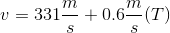, where T is the temperature in °C.

If the sound crew that set up the speakers accidently set up an additional identical speaker directly opposite the base and flipped the phase switch to produce base waves that can be modeled as cosine waves, how would the volume of the sound change at the midpoint of the two speakers?

Possible Answers:

The pitch heard increases

It becomes quieter

It becomes silent

The pitch heard decreases

Correct answer:

It becomes silent

Explanation:

First, let’s look at what this question is asking us to consider. The question wants us to determine the relationship between waves produced from our sine phase base speaker and a cosine phase speaker accidently placed directly across from it. Two important facts come to mind when thinking about this scenario. First, the waves physically overlap in space because the speakers are pointed directly at each other. Second, the sine and cosine waves are exactly 180º out of phase. Remember that waves completely out of phase create destructive interference, meaning that the resultant wave will have no amplitude. The volume directly between the two speakers is zero because volume is directly correlated to wave amplitude.

### Example Question #1 : Interference And Diffraction

Two waves of equal amplitude destructively interfere, resulting in a wave with zero amplitude. What is the phase difference between the two waves?

Possible Answers: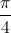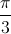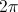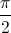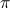Correct answer:Explanation:

Two waves will cancel to zero amplitude when the relative shift between them is half a period. This corresponds to half of, which gives the correct answer of.

### Example Question #3 : Interference And Diffraction

Two people are singing a note at exactly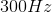. A microphone is placed in front of them to record their voices, but when the track is played back the recorded note is much quieter than the singers were producing. What phenomenon can explain this result?

Possible Answers:

The microphone was unable to pick up the sound because of the large amplitude

The waves went through constructive interference and canceled each other out

The singers' combined frequency was higher than the frequency response of the microphone

The microphone could pick up the same frequency coming from two different sound sources

The waves went through destructive interference and canceled each other out

Correct answer:

The waves went through destructive interference and canceled each other out

Explanation:

Destructive inference occurs when the peak of one wavelength encounters the trough of another wavelength. This causes the waves to cancel each other out, essentially producing a much smaller audible wavelength. In contrast, constructive interference will result in the summation of the wave amplitudes, and an increase in the volume and intensity of the sound.

### Example Question #1 : Interference And Diffraction

A red light (wavelength = 6.5 * 10-7m) shines on a diffraction grating, creating an interference pattern on a distant screen. If a blue light (wavelength= 4.75 * 10-7m) were shone on the same screen instead, the bright lines on the screen would __________.

Possible Answers:

become dimmer

move closer together

move farther apart

become brighter

remain the same

Correct answer:

move closer together

Explanation:

The bright lines from a diffraction grating can be located with the equation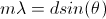, where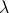is wavelength, d is the distance between slits in the diffraction grating, and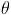is the angle of separation from the center of the interference pattern on the screen.is proportional to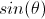, so ifdecreases, the angle of separation between lines also decreases.

### Example Question #1 : Interference And Diffraction

What term describes the phenomenon as a wave spreads into the region behind an obstruction?

Possible Answers:

Diffraction

Superposition

Dispersion

Refraction

Reflection

Correct answer:

Diffraction

Explanation:

Diffraction occurs when a wave passes into a region behind an obstruction (or spreads out when passing through an aperture).

### Example Question #1 : Light

Unpolarized light passes through three polarizing filters. The angle between the first and second filters is 45o. The angle between the second and third filters is also 45o. What is the relationship between the intensity of light emerging from the third filter,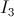, and the incident intensity,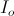?

Possible Answers: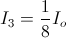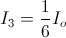No light passes through the third filter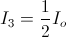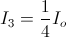Correct answer:Explanation:

Going through the first filter, the intensity of initially unpolarized light decreases by 1/2, so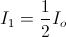. For each filter after the first, the intensity decreases by an additional factor of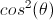, whereis the angle between adjacent polarizers. So, after the second filter, we have can see that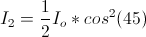. Similarly, after the third filter, we have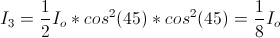.

### Example Question #21 : Waves

Unpolarized monochromatic light passes through a single polaroid filter, oriented horizontally. It then encounters a second filter that is oriented vertically. Which of the following statements is true of the resulting light?

Possible Answers:

The emerging light has one half the incident intensity and is polarized at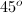The emerging light has the same intensity, but is now polarized atThe emerging light has one-fourth the incident intensity and is polarized vertically

The emerging light has one half of the incident intensity and is polarized vertically

The light is entirely blocked

Correct answer:

The light is entirely blocked

Explanation:

The first filter polarizes the light horizontally, only allowing light to pass if it is oscillating horizontally. This would decrease the intensity by one-half.

The second filter would polarize the light vertically, only allowing light to pass if it oscillates vertically. The first filter, however, has already blocked all non-horizontal waves, including any vertical waves; thus, there are no remaining waves that can pass through the vertical filter. The light is fully blocked by this combination.

### Example Question #1 : Light

Each of the following types of waves experience polarization except __________.

Possible Answers:

sound waves

electromagnetic waves

transverse waves

light waves

Correct answer:

sound waves

Explanation:

Polarization is the property that allows tansverse waves to oscillate in multiple orientations. A transverse wave can oscillate, for example, in either the xy-plane or the yz-plane.

Sound waves are longitudinal, and thus do no experience polarization as medium is displaced in one direction only. A longitudinal wave will travel in only one dimension via compression and rarefraction.

### Example Question #1 : Photons And Photon Energy

The electron shell energies of a particular atom are given below: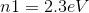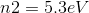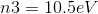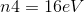Which of the following colors can this atom emit when an electron falls from a higher to lower energy shell?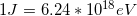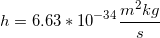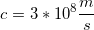Possible Answers:

Red

Yellow

Violet

Green

Orange

Correct answer:

Violet

Explanation:

We can see that eV differences between the shells for this atom range anywhere from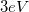(between n2 and n1) to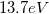(between n4 and n1). We can use these two values to find the range of wavelengths that can be produced by photons from this atom. To find the wavelength, we will use the formula:In this equation,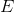is the energy in Joules,is Planck's constant,is the speed of light, and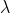is the wavelength.

First, convert the energy changes to Joules using the given conversion factor.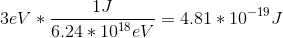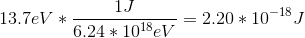Use these energies in the first equation to solve for the range of possible wavelengths.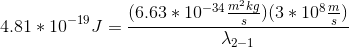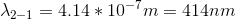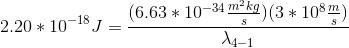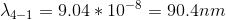The visible spectrum spans from approximately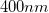to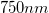, with smaller wavelengths corresponding to violet and blue and larger wavelengths corresponding to red. Since the range of possible wavelengths only overlaps with a small portion of the visible spectrum, we can see that the only color that can be generated when an electron falls from a higher level to a lower level is violet. The other transitions will generate photons in the ultraviolet range.

### Example Question #1 : Light

Light is passed through a prism to separate the wavelengths, and red, green and blue portions of the spectrum are sequentially projected onto a photo-electric surface connected to an electrical circuit. Which of these observations is correct?

1. Red light, but not green or blue light, will generate an electric current.
2. Red light and blue light, but not green light, will generate an electric current.
3. Green light, but not red or blue light, will generate an electric current.
4. Blue light, but not red or green light, will generate an electric current.
5. All the frequencies will generate an electric current if the intensity is adequate.
Possible Answers:

5

3

1

2

4

Correct answer:

4

Explanation:

Choice 4 is correct because blue light is much more energetic than red light. The most intense low-frequency light will not generate any current at all, because the wavelength is not sufficiently energetic to displace electrons from the photo-electric surface; however, once it is established that a certain frequency is capable of generating current, then the amount of current is dependent upon intensity.

Mnemonic:  “Blue is better.”

← Previous 1 3

### All MCAT Physical Resources